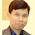# How to Calculate Efficiency of a Production Line?

To know the performance of a garment factory, and a comparative study of the sewing line performance, we measure production line efficiency. So, like individual operator efficiency, measuring the efficiency of a production line is important for a factory. Daily line efficiency shows line performance.

### Production Line Efficiency Calculation - Information required

To calculate the efficiency of a line for a day, you will need the following data (information) from the line supervisor or line recorder.

1. Number of operators – how many operators worked in the line in a day

2. Working hours (Regular and overtime hours) – how many hours each of the operators worked or how many hours the line run in a day

3. Production in pieces – How many pieces are produced or total line output at the end of the day

4. Garment SAM – What is the exact standard minute of the style (garment)

Once you have above data you have to calculate following using above information -
a. Total minutes produced by the line: To get total produced minutes, multiply production pieces by garment SAM
b. Total minutes attended by all operators in the line: Multiply the number of operators by daily working hours and convert total hours into total minutes (multiplying by 60).

Now, calculate line efficiency using the following formula:

Line efficiency (in percentage) = (Total minutes produced by the line *100) /(Total minutes attended by all operators)

### An Example - Calculating Production Line Efficiency

Let's calculate the line efficiency of one production line.
48 operations worked in a line for 8 hours. They produced 160 garments and SAM of the garment is 44.25 minutes.

Here,
Total minutes produced = (160 x 44.25) = 7080 minutes
Total minutes attended = (48 operator x 8 hours x 60) = 23040 minutes
Effiicnecy% = (7080 x100)/23040 % = 30.729%

For more example, refer to the following table. Data calculation formula has been given on the header row of the table.

 No. of Operator (A) Working hours(B) line output (production)(C) Garment SAM(D) Total minutes attended(E=A*B*60) Total Minute produced(F=C*D) Line Efficiency (%)(F/E*100) 48 8 160 44.25 23040 7080 30.73 48 11 240 44.25 31680 10620 33.52 34 8 300 25 16320 7500 45.96 35 11 400 25 23100 10000 43.29 35 11 329 25 23100 8225 35.61 34 8 230 25 16320 5750 35.23 34 8 200 35 16320 7000 42.89 35 11 311 35 23100 10885 47.12 34 11 340 35 22440 11900 53.03

Here is one Video Guide for calculating overall efficiency.

1.1.